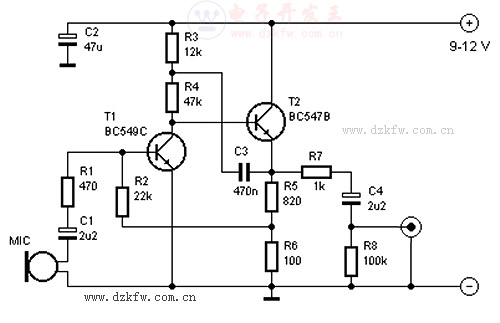# 两个晶体管的麦克风前置放大器，三极管放大电路R1 = 470 Ω
R2 = 22 kΩ
R3 = 12 kΩ
R4 = 47 kΩ
R5 = 820 Ω
R6 = 100 Ω
R7 = 1 kΩ
R8 = 100 kΩ
C1,C4 = 2,2µF/16V
C2 = 47µF/16V
C3 = 470nF
T1 = BC549C
T2 = BC547B

##### Tags：麦克风,前置放大器,放大器,麦克风放大器,麦克风电路

 1分 2分 3分 4分 5分 还可以输入 200 个字

• 此栏目下没有推荐文章